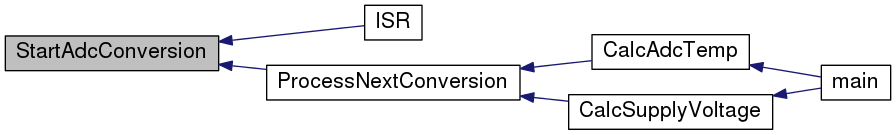heat control  r71
adc.h File Reference
`#include "types.h"`
Include dependency graph for adc.h:This graph shows which files directly or indirectly include this file: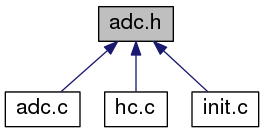Go to the source code of this file.

## Macros

#define ADC_VREF_SEL   ((0<<REFS1) | (0<<REFS0) | (0<<ADLAR))

#define ADC_INPUTS   LAST_ADC_INPUT - FIRST_ADC_INPUT + 1

#define ADC_RES   1024

#define ADC_MEAN_VALUES   50

#define A1   3.354016e-3

#define B1   2.519107e-4

#define C1   3.510939e-6

#define D1   1.105179e-7

## Functions

void AdcInit (void)
initialize the adc resource More...

ISR (ADC_vect)
ADC interrupt service. More...

void ProcessNextConversion (void)
checks whether all converted values are processed and starts a new ADC conversion if the latter evaluates to TRUE More...

void StartAdcConversion (void)
start AD conversion, this will trigger the conversion of all selected channels via the interrupt More...

void SetMux (UCHAR id)
set multiplexer thus switching between channels More...

UINT CalcAdcTemp (UCHAR id)
calculate the temperature from specific ADC value in data array, specified by id More...

UINT CalcSupplyVoltage (UCHAR id)

## Macro Definition Documentation

 #define A1   3.354016e-3

Steinhart-Hart coefficient for temperature calculation

Definition at line 25 of file adc.h.

Referenced by CalcAdcTemp().

 #define ADC_INPUTS   LAST_ADC_INPUT - FIRST_ADC_INPUT + 1

number of adc inputs

Definition at line 13 of file adc.h.

Referenced by ISR().

 #define ADC_MEAN_VALUES   50

number of mean values to form up a valid converted value

Definition at line 21 of file adc.h.

Referenced by CalcAdcTemp(), CalcSupplyVoltage(), and ISR().

 #define ADC_RES   1024

adc resolution

Definition at line 17 of file adc.h.

Referenced by CalcAdcTemp(), and CalcSupplyVoltage().

 #define ADC_VREF_SEL   ((0<

reference voltage selection

Definition at line 9 of file adc.h.

Referenced by SetMux().

 #define B1   2.519107e-4

Steinhart-Hart coefficient for temperature calculation

Definition at line 29 of file adc.h.

Referenced by CalcAdcTemp().

 #define C1   3.510939e-6

Steinhart-Hart coefficient for temperature calculation

Definition at line 33 of file adc.h.

Referenced by CalcAdcTemp().

 #define D1   1.105179e-7

Steinhart-Hart coefficient for temperature calculation

Definition at line 37 of file adc.h.

Referenced by CalcAdcTemp().

## Function Documentation

 void AdcInit ( void )

initialize the adc resource

Definition at line 23 of file adc.c.

References AD_FR, ADC_SR, FIRST_ADC_INPUT, and SetMux().

Referenced by Init().

Here is the call graph for this function: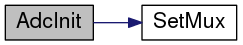Here is the caller graph for this function: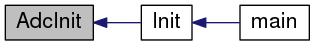UINT CalcAdcTemp ( UCHAR id )

calculate the temperature from specific ADC value in data array, specified by id

Parameters
 id for data array cell to get the temperature for
Returns
calculated temperature according to id

Definition at line 107 of file adc.c.

Referenced by main().

Here is the call graph for this function: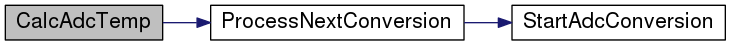Here is the caller graph for this function: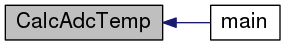UINT CalcSupplyVoltage ( UCHAR id )

Definition at line 126 of file adc.c.

Referenced by main().

Here is the call graph for this function: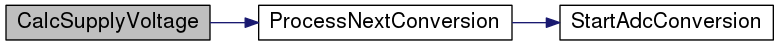Here is the caller graph for this function: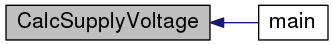ISR ( ADC_vect )

ADC interrupt service.

Parameters
 ADC_vect adc interrupt vector

Definition at line 41 of file adc.c.

Here is the call graph for this function: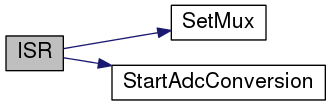void ProcessNextConversion ( void )

checks whether all converted values are processed and starts a new ADC conversion if the latter evaluates to TRUE

Definition at line 74 of file adc.c.

Referenced by CalcAdcTemp(), and CalcSupplyVoltage().

Here is the call graph for this function: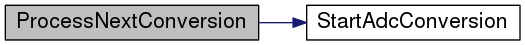Here is the caller graph for this function: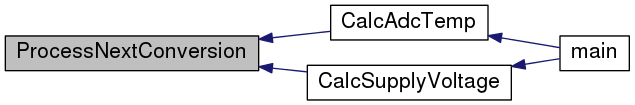void SetMux ( UCHAR ch )

set multiplexer thus switching between channels

Parameters
 ch adc channel id

Definition at line 97 of file adc.c.

References ADC_VREF_SEL, FIRST_ADC_INPUT, g_justMuxed, and TRUE.

Referenced by AdcInit(), and ISR().

Here is the caller graph for this function: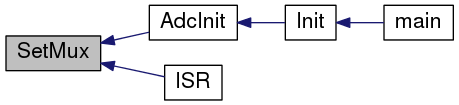void StartAdcConversion ( void )

start AD conversion, this will trigger the conversion of all selected channels via the interrupt

Definition at line 87 of file adc.c.

References ADC_SR, g_adcConverting, and TRUE.

Referenced by ISR(), and ProcessNextConversion().

Here is the caller graph for this function: